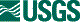## ISIS 2ISIS Application Documentation

# voycal

Printer Friendly View | TOC | Home

Radiometric correction of Voyager 1 and 2 planetary images

 Overview Parameters

## Description

VOYCAL performs radiometric corrections to planetary images acquired by the Viking orbiter and Voyager spacecraft vidicon cameras. VOYCAL performs a radiometric correction in two steps. First, VOYCAL corrects for the varying response of the vidicon across the field-of-view of the camera. Multiplicative and additive correction coefficients, as a function of line and sample position, are applied to an image array to produce the results of an 'ideal' camera. Optionally, for Voyager cameras, a non-linearity correction can be additionally applied to the image data. Secondly, VOYCAL converts the image data to radiance factor values. The radiance factor is defined as the ratio of the observed radiance and the radiance of a white screen, normal to the incident rays of the sun.

EQUATIONS
-----------------

The sensitivity of a vidicon camera varies across the field of view of an image frame. VOYCAL performs an additive, mutiplicative, and non-linearity radiometric correction to an image to correct for the varying sensitivity of the camera. For information on the radiometric properties of a vidicon camera, see the references;

"Inflight Performance of the Viking Visual Imaging Subsystem" Klassen, Thorpe, and Morabito; Applied Optics, Vol. 16, No. 12, December 1977.

"Radiometric Performance of the Voyager Cameras" Danielson, Kuperman, Johnson, and Soderblom; Journal of Geophysical Research, Vol. 86, No. A10, Pages 8683-8689, September 30, 1981.

General equations applied to data for radiometric correction are indicated below:

```Let: i,j    = line and sample position of pixel in an image
DI(i,j) = Result of correction.  This value is known as the
(Radiance factor)*10000. The output of VOYCAL is
defined as the ratio of the observed radiance and
the radiance of a white screen, normal to the incident
rays of the sun. DI(i,j) = 10000 for an ideal 100%
lambertian reflector with the sun and camera orthoganal
to the planet surface.
DR(i,j) = Raw input density number
DC(i,j) = Camera shading dark current. This is a value from a file
G(i,j)  = Camera shading gain. This is a value from a file which
provides the multiplicative correction.
EXP     = Exposure time (seconds)
W0      = Sensitivity of camera with sun-distance correction at
standard distance from the sun.  This value is equivalent
to the density number of a one second exposure of the
camera for the standard distance of the planet from the
sun.
DIST0   = Standard distance of planet.  The W0 value was determined
for a standard planet-sun distance. For Mars, the
standard distance is 1.63 AU, the mean distance Mars from
the Sun.  For Voyager image data, the standard distance
is the distance of the planet (Jupiter, Saturn, Uranus)
at the time of the Voyager encounter.
W1      = Sensitivity of camera with planet-sun distance
correction at actual distance of planet from the sun.
This value is equivalent to the density number of a one
second exposure of the camera for the planet-sun distance.
DIST1   = actual distance of planet from the sun at time image was
recorded
GAIN    = Camera gain state constant
OFF     = Camera state offset condition value

W1 = W0*((DIST0**2)/DIST1**2))

DI(i,j) = (1.0/(EXP*W1))*G(i,j)*(GAIN*DR(i,j)+DC(i,j)+OFF)*10000
```

The linearity correction to the DN values is performed before the gain and offset correction is applied. If the linearity option is chosen, and the voylin.pvl file has a table entry for the mission camera of the data being processed, or the user has provided TAE input for the coefficients B,K,LINORM, then a linearity correction will be made. The result of the linearity correction is fed into the equation shown above. Thus, the result of the linearity correction is called DL(i,j). The following linearity equation is used:

```DL(i,j) = A*(DR(i,j)+DC(i,j)) + B*(((DR(i,j)+DC(i,j))/LINORM)**K)

B = coefficient of linearity
K = power of linearity fit (usually 4)
LINORM = linearizer normalization (usually 128)
A = (LINORM-B)/LINORM
```

## History

 Mackenzie Boyd 2010-08-10 Original version - Converted from Isis2 Debbie A. Cook 2012-07-06 Updated Spice members to be more compliant with Isis coding standards. References #972. Jesse Mapel 2019-08-06 Updated error message when coefficients cannot be found. References #2685.

## Parameter Groups

### Files

Name Description
FROM Input cube for radiometric correction
TO Output cube

### Options

Name Description
LINEAR Option for Linear correction

### Files: FROM

#### Description

The cube to use for radiometric correction, must be a Voyager image.

 Type cube input *.cub

### Files: TO

#### Description

The output cube which will contain the radiometrically corrected data.

 Type cube output real *.cub

### Options: LINEAR

#### Description

Option to apply Linear correction to cube. Must have matching data in voylin.pvl. Equation can be viewed in general description.

 Type boolean TRUE